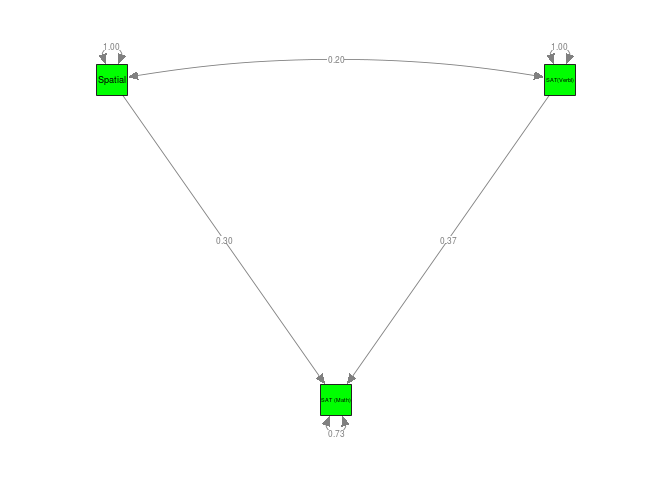# Digman (1997)

## Inspect the data

The correlation matrices and the sample sizes were stored in Digman97$data and Digman97$n, respectively. We may list the first few cases of the data by using the head() command.

  #### Load the metaSEM library for TSSEM
library(metaSEM)

#### Inspect the data for inspection
head(Digman97$data) $Digman 1 (1994)
A     C   ES     E    I
A   1.00  0.62 0.41 -0.48 0.00
C   0.62  1.00 0.59 -0.10 0.35
ES  0.41  0.59 1.00  0.27 0.41
E  -0.48 -0.10 0.27  1.00 0.37
I   0.00  0.35 0.41  0.37 1.00

$Digman 2 (1994) A C ES E I A 1.00 0.39 0.53 -0.30 -0.05 C 0.39 1.00 0.59 0.07 0.44 ES 0.53 0.59 1.00 0.09 0.22 E -0.30 0.07 0.09 1.00 0.45 I -0.05 0.44 0.22 0.45 1.00$Digman 3 (1963c)
A     C   ES     E    I
A  1.00  0.65 0.35  0.25 0.14
C  0.65  1.00 0.37 -0.10 0.33
ES 0.35  0.37 1.00  0.24 0.41
E  0.25 -0.10 0.24  1.00 0.41
I  0.14  0.33 0.41  0.41 1.00

$Digman & Takemoto-Chock (1981b) A C ES E I A 1.00 0.65 0.70 -0.26 -0.03 C 0.65 1.00 0.71 -0.16 0.24 ES 0.70 0.71 1.00 0.01 0.11 E -0.26 -0.16 0.01 1.00 0.66 I -0.03 0.24 0.11 0.66 1.00$Graziano & Ward (1992)
A    C   ES    E    I
A  1.00 0.64 0.35 0.29 0.22
C  0.64 1.00 0.27 0.16 0.22
ES 0.35 0.27 1.00 0.32 0.36
E  0.29 0.16 0.32 1.00 0.53
I  0.22 0.22 0.36 0.53 1.00

$Yik & Bond (1993) A C ES E I A 1.00 0.66 0.57 0.35 0.38 C 0.66 1.00 0.45 0.20 0.31 ES 0.57 0.45 1.00 0.49 0.31 E 0.35 0.20 0.49 1.00 0.59 I 0.38 0.31 0.31 0.59 1.00  head(Digman97$n)
 102 149 334 162  91 656

## Fixed-effects TSSEM

### Stage 1 analysis

To conduct a fixed-effects TSSEM, we may specify method=FEM argument (the default method) in calling the tssem1() function. The results are stored in an object named fixed1. It can be displayed by the summary() command. The $$\chi^2(130, N=4,496) = 1,499.73, p < .001$$, CFI=0.6825, RMSEA=0.1812 and SRMR=0.1750. Based on the test statistic and the goodness-of-fit indices, the assumption of homogeneity of correlation matrices was rejected.

## Fixed-effects model: Stage 1 analysis
fixed1 <- tssem1(my.df=Digman97$data, n=Digman97$n, method="FEM")
summary(fixed1)

Call:
tssem1FEM(my.df = my.df, n = n, cor.analysis = cor.analysis,
model.name = model.name, cluster = cluster, suppressWarnings = suppressWarnings,
silent = silent, run = run)

Coefficients:
Estimate Std.Error z value  Pr(>|z|)
S[1,2] 0.363278  0.013367 27.1764 < 2.2e-16 ***
S[1,3] 0.390375  0.012880 30.3081 < 2.2e-16 ***
S[1,4] 0.103572  0.015047  6.8831 5.858e-12 ***
S[1,5] 0.092286  0.015047  6.1331 8.617e-10 ***
S[2,3] 0.416070  0.012519 33.2349 < 2.2e-16 ***
S[2,4] 0.135148  0.014776  9.1465 < 2.2e-16 ***
S[2,5] 0.141431  0.014866  9.5136 < 2.2e-16 ***
S[3,4] 0.244459  0.014153 17.2725 < 2.2e-16 ***
S[3,5] 0.138339  0.014834  9.3260 < 2.2e-16 ***
S[4,5] 0.424566  0.012376 34.3070 < 2.2e-16 ***
---
Signif. codes:  0 '***' 0.001 '**' 0.01 '*' 0.05 '.' 0.1 ' ' 1

Goodness-of-fit indices:
Value
Sample size                      4496.0000
Chi-square of target model       1505.4443
DF of target model                130.0000
p value of target model             0.0000
Chi-square of independence model 4471.4242
DF of independence model          140.0000
RMSEA                               0.1815
RMSEA lower 95% CI                  0.1736
RMSEA upper 95% CI                  0.1901
SRMR                                0.1621
TLI                                 0.6580
CFI                                 0.6824
AIC                              1245.4443
BIC                               412.0217
OpenMx status1: 0 ("0" or "1": The optimization is considered fine.
Other values may indicate problems.)

The pooled correlation matrix (the parameter estimates) can be extracted by the use of the coef() command.

coef(fixed1)
            A         C        ES         E          I
A  1.00000000 0.3632782 0.3903748 0.1035716 0.09228557
C  0.36327824 1.0000000 0.4160695 0.1351477 0.14143058
ES 0.39037483 0.4160695 1.0000000 0.2444593 0.13833895
E  0.10357155 0.1351477 0.2444593 1.0000000 0.42456626
I  0.09228557 0.1414306 0.1383390 0.4245663 1.00000000

### Stage 2 analysis

As an illustration, I continued to fit the structural model based on the pooled correlation matrix. We may specify the two-factor model with the RAM formulation

## Factor covariance among latent factors
Phi <- matrix(c(1,"0.3*cor","0.3*cor",1), ncol=2, nrow=2)

## Error covariance matrix
Psi <- Diag(c("0.2*e1","0.2*e2","0.2*e3","0.2*e4","0.2*e5"))

## S matrix
S1 <- bdiagMat(list(Psi, Phi))

## This step is not necessary but it is useful for inspecting the model.
dimnames(S1)[] <- dimnames(S1)[] <- c("A","C","ES","E","I","Alpha","Beta")

## Display S1
S1
      A        C        ES       E        I        Alpha     Beta
A     "0.2*e1" "0"      "0"      "0"      "0"      "0"       "0"
C     "0"      "0.2*e2" "0"      "0"      "0"      "0"       "0"
ES    "0"      "0"      "0.2*e3" "0"      "0"      "0"       "0"
E     "0"      "0"      "0"      "0.2*e4" "0"      "0"       "0"
I     "0"      "0"      "0"      "0"      "0.2*e5" "0"       "0"
Alpha "0"      "0"      "0"      "0"      "0"      "1"       "0.3*cor"
Beta  "0"      "0"      "0"      "0"      "0"      "0.3*cor" "1"      
## A matrix
Lambda <-
matrix(c(".3*Alpha_A",".3*Alpha_C",".3*Alpha_ES",rep(0,5),".3*Beta_E",".3*Beta_I"),
ncol=2, nrow=5)
A1 <- rbind( cbind(matrix(0,ncol=5,nrow=5), Lambda),
matrix(0, ncol=7, nrow=2) )

## This step is not necessary but it is useful for inspecting the model.
dimnames(A1)[] <- dimnames(A1)[] <- c("A","C","ES","E","I","Alpha","Beta")

## Display A1
A1
      A   C   ES  E   I   Alpha         Beta
A     "0" "0" "0" "0" "0" ".3*Alpha_A"  "0"
C     "0" "0" "0" "0" "0" ".3*Alpha_C"  "0"
ES    "0" "0" "0" "0" "0" ".3*Alpha_ES" "0"
E     "0" "0" "0" "0" "0" "0"           ".3*Beta_E"
I     "0" "0" "0" "0" "0" "0"           ".3*Beta_I"
Alpha "0" "0" "0" "0" "0" "0"           "0"
Beta  "0" "0" "0" "0" "0" "0"           "0"        
## F matrix to select the observed variables
F1 <- create.Fmatrix(c(1,1,1,1,1,0,0), as.mxMatrix=FALSE)

## Display F1
F1
     [,1] [,2] [,3] [,4] [,5] [,6] [,7]
[1,]    1    0    0    0    0    0    0
[2,]    0    1    0    0    0    0    0
[3,]    0    0    1    0    0    0    0
[4,]    0    0    0    1    0    0    0
[5,]    0    0    0    0    1    0    0

Since we are conducting a correlation structure analysis, the error variances are not free parameters. We need to use the diag.constraints to constrain the diagonals as one. When there are nonlinear constraints, standard errors are not available in OpenMx. We may request the likelihood-based confidence intervals (LBCI) with the intervals argument. The chi-square test statistic of the Stage 2 analysis was $$\chi^2(4, N=4,496) = 65.06, p < .001$$, CFI=0.9802, RMSEA=0.0583 and SRMR=0.0284.

fixed2 <- tssem2(fixed1, Amatrix=A1, Smatrix=S1, Fmatrix=F1,
diag.constraints=TRUE, intervals="LB",
model.name="TSSEM2 Digman97")
summary(fixed2)

Call:
wls(Cov = coef.tssem1FEM(tssem1.obj), asyCov = vcov.tssem1FEM(tssem1.obj),
n = sum(tssem1.obj$n), Amatrix = Amatrix, Smatrix = Smatrix, Fmatrix = Fmatrix, diag.constraints = diag.constraints, cor.analysis = tssem1.obj$cor.analysis,
intervals.type = intervals.type, mx.algebras = mx.algebras,
model.name = model.name, suppressWarnings = suppressWarnings,
silent = silent, run = run)

95% confidence intervals: Likelihood-based statistic
Coefficients:
Estimate Std.Error  lbound  ubound z value Pr(>|z|)
Alpha_A   0.56280        NA 0.53267 0.59302      NA       NA
Alpha_C   0.60522        NA 0.57524 0.63535      NA       NA
Alpha_ES  0.71920        NA 0.68876 0.75032      NA       NA
Beta_E    0.78141        NA 0.71854 0.85503      NA       NA
Beta_I    0.55137        NA 0.49996 0.60273      NA       NA
e1        0.68326        NA 0.64833 0.71623      NA       NA
e2        0.63371        NA 0.59633 0.66912      NA       NA
e3        0.48275        NA 0.43701 0.52563      NA       NA
e4        0.38939        NA 0.26901 0.48349      NA       NA
e5        0.69599        NA 0.63671 0.75022      NA       NA
cor       0.36268        NA 0.31858 0.40646      NA       NA

Goodness-of-fit indices:
Value
Sample size                                4496.0000
Chi-square of target model                   65.4521
DF of target model                            4.0000
p value of target model                       0.0000
Number of constraints imposed on "Smatrix"    5.0000
Chi-square of independence model           3112.9064
DF of independence model                     10.0000
RMSEA                                         0.0585
RMSEA lower 95% CI                            0.0465
RMSEA upper 95% CI                            0.0713
SRMR                                          0.0284
TLI                                           0.9505
CFI                                           0.9802
AIC                                          57.4521
BIC                                          31.8083
OpenMx status1: 0 ("0" or "1": The optimization is considered fine.
Other values indicate problems.)

## Fixed-effects TSSEM with cluster

### Stage 1 analysis

There are 4 types of sample characteristics in the original cluster. We may group them into either younger or older samples.

#### Display the frequencies of "cluster"
table(Digman97$cluster)  Adolescents Children Mature adults Young adults 1 4 6 3  #### Fixed-effects TSSEM with several clusters #### Create a variable for different cluster #### Younger participants: Children and Adolescents #### Older participants: others clusters <- ifelse(Digman97$cluster %in% c("Children","Adolescents"),
yes="Younger participants", no="Older participants")

#### Show the clusters
clusters
  "Younger participants" "Younger participants" "Younger participants"
 "Younger participants" "Younger participants" "Older participants"
 "Older participants"   "Older participants"   "Older participants"
 "Older participants"   "Older participants"   "Older participants"
 "Older participants"   "Older participants"  

We may conduct a fixed-effects model by specifying the cluster=clusters argument. Fixed-effects TSSEM will be conducted according to the labels in the clusters. The goodness-of-fit indices of the Stage 1 analysis for the older and younger participants were $$\chi^2(80, N=3,658) = 823.88, p < .001$$, CFI=0.7437, RMSEA=0.1513 and SRMR=0.1528, and $$\chi^2(40, N=838) = 344.18, p < .001$$, CFI=0.7845, RMSEA=0.2131 and SRMR=0.1508, respectively.

## Example of Fixed-effects TSSEM with several clusters
cluster1 <- tssem1(Digman97$data, Digman97$n, method="FEM",
cluster=clusters)
summary(cluster1)
$Older participants Call: tssem1FEM(my.df = data.cluster[[i]], n = n.cluster[[i]], cor.analysis = cor.analysis, model.name = model.name, suppressWarnings = suppressWarnings) Coefficients: Estimate Std.Error z value Pr(>|z|) S[1,2] 0.297471 0.015436 19.2717 < 2.2e-16 *** S[1,3] 0.370248 0.014532 25.4775 < 2.2e-16 *** S[1,4] 0.137694 0.016403 8.3944 < 2.2e-16 *** S[1,5] 0.098061 0.016724 5.8636 4.528e-09 *** S[2,3] 0.393692 0.014146 27.8308 < 2.2e-16 *** S[2,4] 0.183045 0.016055 11.4009 < 2.2e-16 *** S[2,5] 0.092774 0.016643 5.5743 2.485e-08 *** S[3,4] 0.260753 0.015554 16.7645 < 2.2e-16 *** S[3,5] 0.096141 0.016574 5.8009 6.597e-09 *** S[4,5] 0.411756 0.013900 29.6225 < 2.2e-16 *** --- Signif. codes: 0 '***' 0.001 '**' 0.01 '*' 0.05 '.' 0.1 ' ' 1 Goodness-of-fit indices: Value Sample size 3658.0000 Chi-square of target model 825.9826 DF of target model 80.0000 p value of target model 0.0000 Chi-square of independence model 3000.9731 DF of independence model 90.0000 RMSEA 0.1515 RMSEA lower 95% CI 0.1424 RMSEA upper 95% CI 0.1611 SRMR 0.1459 TLI 0.7117 CFI 0.7437 AIC 665.9826 BIC 169.6088 OpenMx status1: 0 ("0" or "1": The optimization is considered fine. Other values may indicate problems.)$Younger participants

Call:
tssem1FEM(my.df = data.cluster[[i]], n = n.cluster[[i]], cor.analysis = cor.analysis,
model.name = model.name, suppressWarnings = suppressWarnings)

Coefficients:
Estimate Std.Error z value  Pr(>|z|)
S[1,2]  0.604330  0.022125 27.3138 < 2.2e-16 ***
S[1,3]  0.465536  0.027493 16.9326 < 2.2e-16 ***
S[1,4] -0.031381  0.035940 -0.8731   0.38259
S[1,5]  0.061508  0.034547  1.7804   0.07501 .
S[2,3]  0.501432  0.026348 19.0309 < 2.2e-16 ***
S[2,4] -0.060678  0.034558 -1.7559   0.07911 .
S[2,5]  0.320987  0.031064 10.3329 < 2.2e-16 ***
S[3,4]  0.175437  0.033675  5.2097 1.892e-07 ***
S[3,5]  0.305149  0.031586  9.6608 < 2.2e-16 ***
S[4,5]  0.478640  0.026883 17.8045 < 2.2e-16 ***
---
Signif. codes:  0 '***' 0.001 '**' 0.01 '*' 0.05 '.' 0.1 ' ' 1

Goodness-of-fit indices:
Value
Sample size                       838.0000
Chi-square of target model        346.2810
DF of target model                 40.0000
p value of target model             0.0000
Chi-square of independence model 1470.4511
DF of independence model           50.0000
RMSEA                               0.2139
RMSEA lower 95% CI                  0.1939
RMSEA upper 95% CI                  0.2355
SRMR                                0.1411
TLI                                 0.7305
CFI                                 0.7844
AIC                               266.2810
BIC                                77.0402
OpenMx status1: 0 ("0" or "1": The optimization is considered fine.
Other values may indicate problems.)

The pooled correlation matrix for each cluster can be extracted by the use of the =coef()= command.

coef(cluster1)
$Older participants A C ES E I A 1.00000000 0.29747123 0.37024803 0.1376942 0.09806125 C 0.29747123 1.00000000 0.39369157 0.1830450 0.09277411 ES 0.37024803 0.39369157 1.00000000 0.2607530 0.09614072 E 0.13769424 0.18304500 0.26075304 1.0000000 0.41175622 I 0.09806125 0.09277411 0.09614072 0.4117562 1.00000000$Younger participants
A          C        ES          E          I
A   1.00000000  0.6043300 0.4655359 -0.0313810 0.06150839
C   0.60433002  1.0000000 0.5014319 -0.0606784 0.32098713
ES  0.46553592  0.5014319 1.0000000  0.1754367 0.30514853
E  -0.03138100 -0.0606784 0.1754367  1.0000000 0.47864004
I   0.06150839  0.3209871 0.3051485  0.4786400 1.00000000

### Stage 2 analysis

The goodness-of-fit indices of the Stage 2 analysis for the older and younger participants were $$\chi^2(4, N=3,658) = 21.92, p < .001$$, CFI=0.9921, RMSEA=0.0350 and SRMR=0.0160, and $$\chi^2(4, N=838) = 144.87, p < .001$$, CFI=0.9427, RMSEA=0.2051 and SRMR=0.1051, respectively.

cluster2 <- tssem2(cluster1, Amatrix=A1, Smatrix=S1, Fmatrix=F1,
diag.constraints=TRUE, intervals.type="z")
#### Please note that the estimates for the younger participants are problematic.
summary(cluster2)
Warning in vcov.wls(object, R = R): Parametric bootstrap with 50 replications was used to approximate the sampling covariance matrix of the parameter estimates. A better approach is to use likelihood-based confidence interval by including the intervals.type="LB" argument in the analysis.

Warning in vcov.wls(object, R = R): Parametric bootstrap with 50 replications was used to approximate the sampling covariance matrix of the parameter estimates. A better approach is to use likelihood-based confidence interval by including the intervals.type="LB" argument in the analysis.
$Older participants Call: wls(Cov = coef.tssem1FEM(tssem1.obj), asyCov = vcov.tssem1FEM(tssem1.obj), n = sum(tssem1.obj$n), Amatrix = Amatrix, Smatrix = Smatrix,
Fmatrix = Fmatrix, diag.constraints = diag.constraints, cor.analysis = tssem1.obj$cor.analysis, intervals.type = intervals.type, mx.algebras = mx.algebras, model.name = model.name, suppressWarnings = suppressWarnings, silent = silent, run = run) 95% confidence intervals: z statistic approximation Coefficients: Estimate Std.Error lbound ubound z value Pr(>|z|) Alpha_A 0.512656 0.020807 0.471875 0.553437 24.6387 <2e-16 *** Alpha_C 0.549967 0.020503 0.509782 0.590152 26.8238 <2e-16 *** Alpha_ES 0.732173 0.016625 0.699590 0.764757 44.0415 <2e-16 *** Beta_E 0.967137 0.066308 0.837175 1.097098 14.5855 <2e-16 *** Beta_I 0.430648 0.030387 0.371091 0.490206 14.1721 <2e-16 *** e1 0.737184 0.021255 0.695525 0.778843 34.6827 <2e-16 *** e2 0.697536 0.022287 0.653854 0.741219 31.2974 <2e-16 *** e3 0.463922 0.024632 0.415645 0.512199 18.8345 <2e-16 *** e4 0.064646 0.132357 -0.194769 0.324062 0.4884 0.6253 e5 0.814542 0.025836 0.763905 0.865179 31.5278 <2e-16 *** cor 0.349235 0.027080 0.296160 0.402310 12.8966 <2e-16 *** --- Signif. codes: 0 '***' 0.001 '**' 0.01 '*' 0.05 '.' 0.1 ' ' 1 Goodness-of-fit indices: Value Sample size 3658.0000 Chi-square of target model 21.9955 DF of target model 4.0000 p value of target model 0.0002 Number of constraints imposed on "Smatrix" 5.0000 DF manually adjusted 0.0000 Chi-square of independence model 2273.3348 DF of independence model 10.0000 RMSEA 0.0351 RMSEA lower 95% CI 0.0217 RMSEA upper 95% CI 0.0500 SRMR 0.0160 TLI 0.9801 CFI 0.9920 AIC 13.9955 BIC -10.8232 OpenMx status1: 0 ("0" or "1": The optimization is considered fine. Other values indicate problems.)$Younger participants

Call:
wls(Cov = coef.tssem1FEM(tssem1.obj), asyCov = vcov.tssem1FEM(tssem1.obj),
n = sum(tssem1.obj$n), Amatrix = Amatrix, Smatrix = Smatrix, Fmatrix = Fmatrix, diag.constraints = diag.constraints, cor.analysis = tssem1.obj$cor.analysis,
intervals.type = intervals.type, mx.algebras = mx.algebras,
model.name = model.name, suppressWarnings = suppressWarnings,
silent = silent, run = run)

95% confidence intervals: z statistic approximation
Coefficients:
Estimate   Std.Error      lbound      ubound z value  Pr(>|z|)
Alpha_A   7.4765e-01  3.0120e-02  6.8861e-01  8.0668e-01 24.8224 < 2.2e-16
Alpha_C   9.1170e-01  2.0758e-02  8.7102e-01  9.5239e-01 43.9205 < 2.2e-16
Alpha_ES  6.7744e-01  2.7205e-02  6.2411e-01  7.3076e-01 24.9008 < 2.2e-16
Beta_E    1.5258e-01  1.1829e-01 -7.9271e-02  3.8443e-01  1.2899    0.1971
Beta_I    3.2834e+00  2.1744e+02 -4.2288e+02  4.2945e+02  0.0151    0.9880
e1        4.4102e-01  4.5340e-02  3.5216e-01  5.2989e-01  9.7269 < 2.2e-16
e2        1.6879e-01  3.7936e-02  9.4441e-02  2.4315e-01  4.4495 8.608e-06
e3        5.4108e-01  3.6878e-02  4.6880e-01  6.1336e-01 14.6720 < 2.2e-16
e4        9.7672e-01  3.6639e-02  9.0491e-01  1.0485e+00 26.6577 < 2.2e-16
e5       -9.7810e+00  1.2346e+05 -2.4199e+05  2.4197e+05 -0.0001    0.9999
cor       1.1727e-01  9.0083e-02 -5.9287e-02  2.9383e-01  1.3018    0.1930

Alpha_A  ***
Alpha_C  ***
Alpha_ES ***
Beta_E
Beta_I
e1       ***
e2       ***
e3       ***
e4       ***
e5
cor
---
Signif. codes:  0 '***' 0.001 '**' 0.01 '*' 0.05 '.' 0.1 ' ' 1

Goodness-of-fit indices:
Value
Sample size                                 838.0000
Chi-square of target model                  145.6174
DF of target model                            4.0000
p value of target model                       0.0000
Number of constraints imposed on "Smatrix"    5.0000
Chi-square of independence model           2480.1661
DF of independence model                     10.0000
RMSEA                                         0.2057
RMSEA lower 95% CI                            0.1778
RMSEA upper 95% CI                            0.2350
SRMR                                          0.1051
TLI                                           0.8567
CFI                                           0.9427
AIC                                         137.6174
BIC                                         118.6933
OpenMx status1: 0 ("0" or "1": The optimization is considered fine.
Other values indicate problems.)

We may also plot the parameter estimates of these two groups.

library(semPlot)

## Convert the model to semPlotModel object with 2 plots
my.plots <- lapply(X=cluster2, FUN=meta2semPlot, latNames=c("Alpha","Beta"))

## Setup two plots
layout(t(1:2))
semPaths(my.plots[], whatLabels="est", nCharNodes=10, color="green")
title("Younger")
semPaths(my.plots[], whatLabels="est", nCharNodes=10, color="yellow")
title("Older")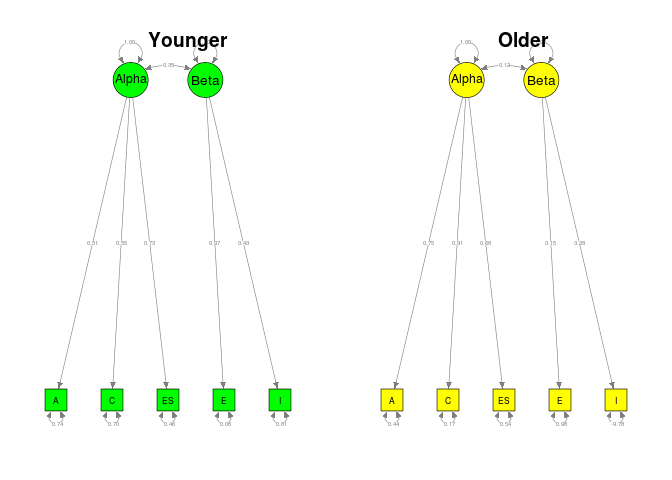## Random-effects TSSEM

### Stage 1 analysis

Since there is not enough data to estimate the full variance component of the random effects, I estimate the variance component with a diagonal matrix in the RE.type="Diag" argument. The range of $$I^2$$ indices, the percentage of total variance that can be explained by the between study effect, are from .84 to .95.

#### Random-effects TSSEM with random effects on the diagonals
random1 <- tssem1(Digman97$data, Digman97$n, method="REM",
RE.type="Diag")
summary(random1)

Call:
meta(y = ES, v = acovR, RE.constraints = Diag(x = paste(RE.startvalues,
"*Tau2_", 1:no.es, "_", 1:no.es, sep = "")), RE.lbound = RE.lbound,
I2 = I2, model.name = model.name, suppressWarnings = TRUE,
silent = silent, run = run)

95% confidence intervals: z statistic approximation
Coefficients:
Estimate   Std.Error      lbound      ubound z value
Intercept1   3.9460e-01  5.4212e-02  2.8835e-01  5.0085e-01  7.2789
Intercept2   4.4005e-01  4.1241e-02  3.5922e-01  5.2088e-01 10.6701
Intercept3   5.4509e-02  6.1730e-02 -6.6479e-02  1.7550e-01  0.8830
Intercept4   9.8644e-02  4.6202e-02  8.0884e-03  1.8920e-01  2.1350
Intercept5   4.2958e-01  4.0146e-02  3.5090e-01  5.0827e-01 10.7004
Intercept6   1.2851e-01  4.0839e-02  4.8472e-02  2.0856e-01  3.1469
Intercept7   2.0521e-01  4.9554e-02  1.0809e-01  3.0233e-01  4.1411
Intercept8   2.3988e-01  3.1927e-02  1.7730e-01  3.0245e-01  7.5134
Intercept9   1.8907e-01  4.2998e-02  1.0480e-01  2.7335e-01  4.3972
Intercept10  4.4403e-01  3.2524e-02  3.8029e-01  5.0778e-01 13.6524
Tau2_1_1     3.7222e-02  1.5000e-02  7.8227e-03  6.6621e-02  2.4815
Tau2_2_2     2.0313e-02  8.4334e-03  3.7838e-03  3.6842e-02  2.4086
Tau2_3_3     4.8285e-02  1.9739e-02  9.5972e-03  8.6972e-02  2.4462
Tau2_4_4     2.4629e-02  1.0625e-02  3.8049e-03  4.5453e-02  2.3181
Tau2_5_5     1.8744e-02  8.2474e-03  2.5796e-03  3.4909e-02  2.2727
Tau2_6_6     1.8317e-02  8.8016e-03  1.0660e-03  3.5568e-02  2.0811
Tau2_7_7     2.9412e-02  1.2252e-02  5.3988e-03  5.3424e-02  2.4006
Tau2_8_8     9.6824e-03  4.8934e-03  9.1508e-05  1.9273e-02  1.9787
Tau2_9_9     2.0950e-02  9.1284e-03  3.0588e-03  3.8842e-02  2.2951
Tau2_10_10   1.1156e-02  5.0453e-03  1.2672e-03  2.1044e-02  2.2111
Pr(>|z|)
Intercept1  3.366e-13 ***
Intercept2  < 2.2e-16 ***
Intercept3    0.37722
Intercept4    0.03276 *
Intercept5  < 2.2e-16 ***
Intercept6    0.00165 **
Intercept7  3.456e-05 ***
Intercept8  5.751e-14 ***
Intercept9  1.097e-05 ***
Intercept10 < 2.2e-16 ***
Tau2_1_1      0.01308 *
Tau2_2_2      0.01601 *
Tau2_3_3      0.01444 *
Tau2_4_4      0.02044 *
Tau2_5_5      0.02304 *
Tau2_6_6      0.03743 *
Tau2_7_7      0.01637 *
Tau2_8_8      0.04785 *
Tau2_9_9      0.02173 *
Tau2_10_10    0.02703 *
---
Signif. codes:  0 '***' 0.001 '**' 0.01 '*' 0.05 '.' 0.1 ' ' 1

Q statistic on the homogeneity of effect sizes: 2390.052
Degrees of freedom of the Q statistic: 130
P value of the Q statistic: 0

Heterogeneity indices (based on the estimated Tau2):
Estimate
Intercept1: I2 (Q statistic)    0.9489
Intercept2: I2 (Q statistic)    0.9085
Intercept3: I2 (Q statistic)    0.9417
Intercept4: I2 (Q statistic)    0.8898
Intercept5: I2 (Q statistic)    0.9009
Intercept6: I2 (Q statistic)    0.8545
Intercept7: I2 (Q statistic)    0.9096
Intercept8: I2 (Q statistic)    0.7726
Intercept9: I2 (Q statistic)    0.8751
Intercept10: I2 (Q statistic)   0.8437

Number of studies (or clusters): 14
Number of observed statistics: 140
Number of estimated parameters: 20
Degrees of freedom: 120
-2 log likelihood: -110.8764
OpenMx status1: 0 ("0" or "1": The optimization is considered fine.
Other values may indicate problems.)

The pooled correlation coefficients (fixed effects) and the variance components (the random effects) can be extracted by the use of the coef() command via the select argument.

## Fixed effects
coef(random1, select="fixed")
 Intercept1  Intercept2  Intercept3  Intercept4  Intercept5  Intercept6
0.39459999  0.44005201  0.05450942  0.09864354  0.42958326  0.12851430
Intercept7  Intercept8  Intercept9 Intercept10
0.20521009  0.23987945  0.18907142  0.44403320 
## Random effects
coef(random1, select="random")
   Tau2_1_1    Tau2_2_2    Tau2_3_3    Tau2_4_4    Tau2_5_5    Tau2_6_6
0.037222059 0.020313026 0.048284535 0.024629148 0.018744111 0.018316939
Tau2_7_7    Tau2_8_8    Tau2_9_9  Tau2_10_10
0.029411543 0.009682373 0.020950191 0.011155798 

### Stage 2 analysis

The steps are exactly the same as those in the fixed-effects model. The chi-square test statistic of the Stage 2 analysis was $$\chi^2(4, N=4,496) = 8.51, p < .001$$, CFI=0.9911, RMSEA=0.0158 and SRMR=0.0463.

random2 <- tssem2(random1, Amatrix=A1, Smatrix=S1, Fmatrix=F1,
diag.constraints=TRUE, intervals="LB")
summary(random2)

Call:
wls(Cov = pooledS, asyCov = asyCov, n = tssem1.obj$total.n, Amatrix = Amatrix, Smatrix = Smatrix, Fmatrix = Fmatrix, diag.constraints = diag.constraints, cor.analysis = cor.analysis, intervals.type = intervals.type, mx.algebras = mx.algebras, model.name = model.name, suppressWarnings = suppressWarnings, silent = silent, run = run) 95% confidence intervals: Likelihood-based statistic Coefficients: Estimate Std.Error lbound ubound z value Pr(>|z|) Alpha_A 0.57255 NA 0.47380 0.67681 NA NA Alpha_C 0.59013 NA 0.49058 0.69480 NA NA Alpha_ES 0.77030 NA 0.65984 0.79384 NA NA Beta_E 0.69329 NA 0.56260 NA NA NA Beta_I 0.64007 NA 0.50847 0.78612 NA NA e1 0.67218 NA 0.54195 0.77555 NA NA e2 0.65175 NA 0.51718 0.75934 NA NA e3 0.40664 NA 0.18850 0.56458 NA NA e4 0.51935 NA 0.40569 0.68393 NA NA e5 0.59031 NA 0.46535 0.74156 NA NA cor 0.39366 NA 0.30246 0.49017 NA NA Goodness-of-fit indices: Value Sample size 4496.0000 Chi-square of target model 8.5069 DF of target model 4.0000 p value of target model 0.0747 Number of constraints imposed on "Smatrix" 5.0000 DF manually adjusted 0.0000 Chi-square of independence model 515.0233 DF of independence model 10.0000 RMSEA 0.0158 RMSEA lower 95% CI 0.0000 RMSEA upper 95% CI 0.0308 SRMR 0.0463 TLI 0.9777 CFI 0.9911 AIC 0.5069 BIC -25.1369 OpenMx status1: 0 ("0" or "1": The optimization is considered fine. Other values indicate problems.) We may also plot the models with the labels or the parameter estimates. library(semPlot) ## Convert the model to semPlotModel object my.plot <- meta2semPlot(random2, latNames=c("Alpha","Beta")) ## Plot the model with labels semPaths(my.plot, whatLabels="path", nCharEdges=10, nCharNodes=10, color="red")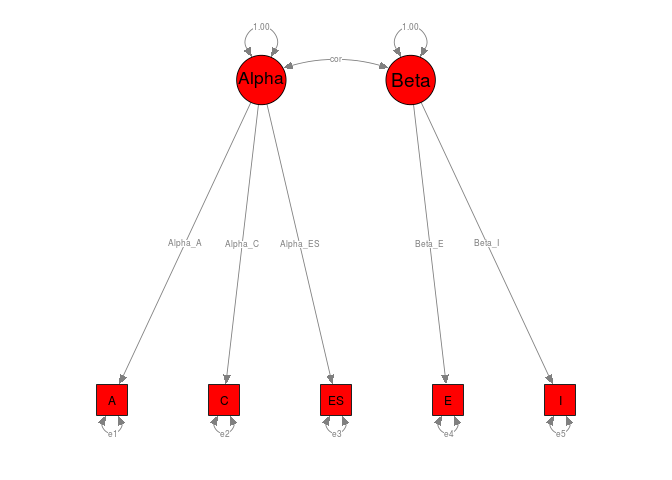## Plot the parameter estimates semPaths(my.plot, whatLabels="est", nCharNodes=10, color="green")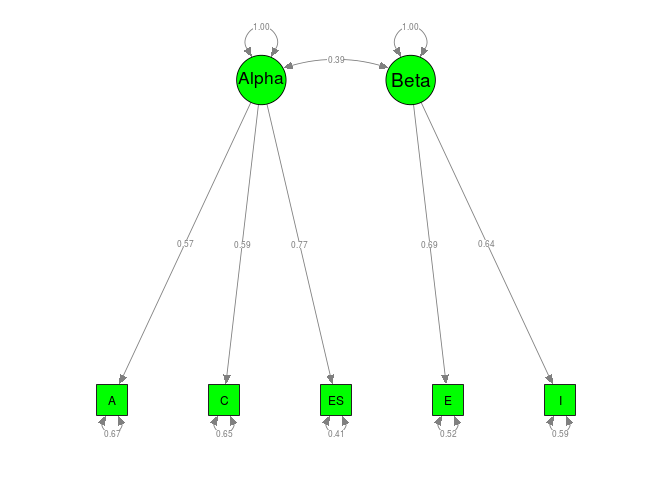# Becker and Schram (1994) ## Inspect the data We may list the first few cases of the data by using the head() command. #### Load the metaSEM library for TSSEM library(metaSEM) #### Inspect the data for inspection (not required for the analysis) head(Becker94$data)
$Becker (1978) Females SAT (Math) Spatial SAT (Verbal) SAT (Math) 1.00 0.47 -0.21 Spatial 0.47 1.00 -0.15 SAT (Verbal) -0.21 -0.15 1.00$Becker (1978) Males
SAT (Math) Spatial SAT (Verbal)
SAT (Math)         1.00    0.28         0.19
Spatial            0.28    1.00         0.18
SAT (Verbal)       0.19    0.18         1.00

$Berry (1957) Females SAT (Math) Spatial SAT (Verbal) SAT (Math) 1.00 0.48 0.41 Spatial 0.48 1.00 0.26 SAT (Verbal) 0.41 0.26 1.00$Berry (1957) Males
SAT (Math) Spatial SAT (Verbal)
SAT (Math)         1.00    0.37         0.40
Spatial            0.37    1.00         0.27
SAT (Verbal)       0.40    0.27         1.00

$Rosenberg (1981) Females SAT (Math) Spatial SAT (Verbal) SAT (Math) 1.00 0.42 0.48 Spatial 0.42 1.00 0.23 SAT (Verbal) 0.48 0.23 1.00$Rosenberg (1981) Males
SAT (Math) Spatial SAT (Verbal)
SAT (Math)         1.00    0.41         0.74
Spatial            0.41    1.00         0.44
SAT (Verbal)       0.74    0.44         1.00
head(Becker94$n)  74 153 48 55 51 18 ## Fixed-effects TSSEM ### Stage 1 analysis The test statistic of the Stage 1 analysis based on the TSSEM approach was $$\chi^2(27, N=538) = 62.50, p < .001$$, CFI=0.7943, RMSEA=0.1565 and SRMR=0.2011. Based on the test statistic and the goodness-of-fit indices, the hypothesis of homogeneity of correlation matrices was rejected. #### Fixed-effects model ## Stage 1 analysis fixed1 <- tssem1(Becker94$data, Becker94$n, method="FEM") summary(fixed1)  Call: tssem1FEM(my.df = my.df, n = n, cor.analysis = cor.analysis, model.name = model.name, cluster = cluster, suppressWarnings = suppressWarnings, silent = silent, run = run) Coefficients: Estimate Std.Error z value Pr(>|z|) S[1,2] 0.379961 0.037124 10.2350 < 2.2e-16 *** S[1,3] 0.334562 0.039947 8.3751 < 2.2e-16 *** S[2,3] 0.176461 0.042334 4.1683 3.069e-05 *** --- Signif. codes: 0 '***' 0.001 '**' 0.01 '*' 0.05 '.' 0.1 ' ' 1 Goodness-of-fit indices: Value Sample size 538.0000 Chi-square of target model 63.6553 DF of target model 27.0000 p value of target model 0.0001 Chi-square of independence model 207.7894 DF of independence model 30.0000 RMSEA 0.1590 RMSEA lower 95% CI 0.1096 RMSEA upper 95% CI 0.2117 SRMR 0.1586 TLI 0.7709 CFI 0.7938 AIC 9.6553 BIC -106.1169 OpenMx status1: 0 ("0" or "1": The optimization is considered fine. Other values may indicate problems.) coef(fixed1)  SAT (Math) Spatial SAT (Verbal) SAT (Math) 1.0000000 0.3799605 0.334562 Spatial 0.3799605 1.0000000 0.176461 SAT (Verbal) 0.3345620 0.1764610 1.000000 ### Stage 2 analysis We may specify the model via the RAM formulation. If all variables are observed, there is no need to specify the F matrix. Since the df of the regression model is 0, the proposed model always fits the data perfectly. #### Prepare models for stage 2 analysis ## A1: asymmetric matrix (regression coefficients) A1 <- create.mxMatrix(c(0,0,0,"0.2*Spatial2Math", 0,0,"0.2*Verbal2Math",0,0), type="Full", ncol=3, nrow=3, name="A1") A1  FullMatrix 'A1'$labels
[,1] [,2]           [,3]
[1,] NA   "Spatial2Math" "Verbal2Math"
[2,] NA   NA             NA
[3,] NA   NA             NA

$values [,1] [,2] [,3] [1,] 0 0.2 0.2 [2,] 0 0.0 0.0 [3,] 0 0.0 0.0$free
[,1]  [,2]  [,3]
[1,] FALSE  TRUE  TRUE
[2,] FALSE FALSE FALSE
[3,] FALSE FALSE FALSE

$lbound: No lower bounds assigned.$ubound: No upper bounds assigned.
## S1: symmetric matrix (variance covariance matrix among variables)
S1 <- create.mxMatrix(c("0.2*ErrorVarMath",0,0,1,"0.2*CorBetweenSpatialVerbal",1),
type="Symm", name="S1")
S1
SymmMatrix 'S1'

$labels [,1] [,2] [,3] [1,] "ErrorVarMath" NA NA [2,] NA NA "CorBetweenSpatialVerbal" [3,] NA "CorBetweenSpatialVerbal" NA$values
[,1] [,2] [,3]
[1,]  0.2  0.0  0.0
[2,]  0.0  1.0  0.2
[3,]  0.0  0.2  1.0

$free [,1] [,2] [,3] [1,] TRUE FALSE FALSE [2,] FALSE FALSE TRUE [3,] FALSE TRUE FALSE$lbound: No lower bounds assigned.

$ubound: No upper bounds assigned. ## Stage 2 analysis fixed2 <- tssem2(fixed1, Amatrix=A1, Smatrix=S1, diag.constraints=TRUE, intervals="LB") summary(fixed2)  Call: wls(Cov = coef.tssem1FEM(tssem1.obj), asyCov = vcov.tssem1FEM(tssem1.obj), n = sum(tssem1.obj$n), Amatrix = Amatrix, Smatrix = Smatrix,
Fmatrix = Fmatrix, diag.constraints = diag.constraints, cor.analysis = tssem1.obj$cor.analysis, intervals.type = intervals.type, mx.algebras = mx.algebras, model.name = model.name, suppressWarnings = suppressWarnings, silent = silent, run = run) 95% confidence intervals: Likelihood-based statistic Coefficients: Estimate Std.Error lbound ubound z value Spatial2Math 0.331238 NA 0.258215 0.404149 NA Verbal2Math 0.276111 NA 0.199500 0.352777 NA ErrorVarMath 0.781766 NA 0.714803 0.840626 NA CorBetweenSpatialVerbal 0.176461 NA 0.093482 0.259435 NA Pr(>|z|) Spatial2Math NA Verbal2Math NA ErrorVarMath NA CorBetweenSpatialVerbal NA Goodness-of-fit indices: Value Sample size 538.00 Chi-square of target model 0.00 DF of target model 0.00 p value of target model 0.00 Number of constraints imposed on "Smatrix" 1.00 DF manually adjusted 0.00 Chi-square of independence model 160.47 DF of independence model 3.00 RMSEA 0.00 RMSEA lower 95% CI 0.00 RMSEA upper 95% CI 0.00 SRMR 0.00 TLI -Inf CFI 1.00 AIC 0.00 BIC 0.00 OpenMx status1: 0 ("0" or "1": The optimization is considered fine. Other values indicate problems.) ## Fixed-effects TSSEM with cluster ### Stage 1 analysis The goodness-of-fit indices of the Stage 1 analysis for the female and male participants were $$\chi^2(12, N=235) = 42.41, p < .001$$, CFI=0.7116, RMSEA=0.2327 and SRMR=0.2339, and $$\chi^2(12, N=303) = 16.13, p = .1852$$, CFI=0.9385, RMSEA=0.0755 and SRMR=0.1508, respectively. #### Fixed-effects model with cluster ## Stage 1 analysis cluster1 <- tssem1(Becker94$data, Becker94$n, method="FEM", cluster=Becker94$gender)
summary(cluster1)
$Females Call: tssem1FEM(my.df = data.cluster[[i]], n = n.cluster[[i]], cor.analysis = cor.analysis, model.name = model.name, suppressWarnings = suppressWarnings) Coefficients: Estimate Std.Error z value Pr(>|z|) S[1,2] 0.455896 0.051992 8.7685 < 2.2e-16 *** S[1,3] 0.341583 0.061943 5.5144 3.499e-08 *** S[2,3] 0.171931 0.064731 2.6561 0.007905 ** --- Signif. codes: 0 '***' 0.001 '**' 0.01 '*' 0.05 '.' 0.1 ' ' 1 Goodness-of-fit indices: Value Sample size 235.0000 Chi-square of target model 43.1898 DF of target model 12.0000 p value of target model 0.0000 Chi-square of independence model 123.4399 DF of independence model 15.0000 RMSEA 0.2357 RMSEA lower 95% CI 0.1637 RMSEA upper 95% CI 0.3161 SRMR 0.2141 TLI 0.6405 CFI 0.7124 AIC 19.1898 BIC -22.3252 OpenMx status1: 0 ("0" or "1": The optimization is considered fine. Other values may indicate problems.)$Males

Call:
tssem1FEM(my.df = data.cluster[[i]], n = n.cluster[[i]], cor.analysis = cor.analysis,
model.name = model.name, suppressWarnings = suppressWarnings)

Coefficients:
Estimate Std.Error z value  Pr(>|z|)
S[1,2] 0.318051  0.051698  6.1521 7.646e-10 ***
S[1,3] 0.328286  0.052226  6.2859 3.261e-10 ***
S[2,3] 0.179549  0.055944  3.2094   0.00133 **
---
Signif. codes:  0 '***' 0.001 '**' 0.01 '*' 0.05 '.' 0.1 ' ' 1

Goodness-of-fit indices:
Value
Sample size                      303.0000
Chi-square of target model        16.4819
DF of target model                12.0000
p value of target model            0.1701
Chi-square of independence model  84.3496
DF of independence model          15.0000
RMSEA                              0.0786
RMSEA lower 95% CI                 0.0000
RMSEA upper 95% CI                 0.1643
SRMR                               0.1025
TLI                                0.9192
CFI                                0.9354
AIC                               -7.5181
BIC                              -52.0829
OpenMx status1: 0 ("0" or "1": The optimization is considered fine.
Other values may indicate problems.)
coef(cluster1)
$Females SAT (Math) Spatial SAT (Verbal) SAT (Math) 1.0000000 0.4558958 0.3415826 Spatial 0.4558958 1.0000000 0.1719309 SAT (Verbal) 0.3415826 0.1719309 1.0000000$Males
SAT (Math)   Spatial SAT (Verbal)
SAT (Math)    1.0000000 0.3180507    0.3282856
Spatial       0.3180507 1.0000000    0.1795489
SAT (Verbal)  0.3282856 0.1795489    1.0000000

### Stage 2 analysis

## Stage 2 analysis
cluster2 <- tssem2(cluster1, Amatrix=A1, Smatrix=S1, diag.constraints=TRUE,
intervals="LB")
summary(cluster2)
$Females Call: wls(Cov = coef.tssem1FEM(tssem1.obj), asyCov = vcov.tssem1FEM(tssem1.obj), n = sum(tssem1.obj$n), Amatrix = Amatrix, Smatrix = Smatrix,
Fmatrix = Fmatrix, diag.constraints = diag.constraints, cor.analysis = tssem1.obj$cor.analysis, intervals.type = intervals.type, mx.algebras = mx.algebras, model.name = model.name, suppressWarnings = suppressWarnings, silent = silent, run = run) 95% confidence intervals: Likelihood-based statistic Coefficients: Estimate Std.Error lbound ubound z value Spatial2Math 0.409265 NA 0.305287 0.512539 NA Verbal2Math 0.271217 NA 0.156124 0.386562 NA ErrorVarMath 0.720775 NA 0.613550 0.812622 NA CorBetweenSpatialVerbal 0.171931 NA 0.045058 0.298801 NA Pr(>|z|) Spatial2Math NA Verbal2Math NA ErrorVarMath NA CorBetweenSpatialVerbal NA Goodness-of-fit indices: Value Sample size 235.00 Chi-square of target model 0.00 DF of target model 0.00 p value of target model 0.00 Number of constraints imposed on "Smatrix" 1.00 DF manually adjusted 0.00 Chi-square of independence model 105.57 DF of independence model 3.00 RMSEA 0.00 RMSEA lower 95% CI 0.00 RMSEA upper 95% CI 0.00 SRMR 0.00 TLI -Inf CFI 1.00 AIC 0.00 BIC 0.00 OpenMx status1: 0 ("0" or "1": The optimization is considered fine. Other values indicate problems.)$Males

Call:
wls(Cov = coef.tssem1FEM(tssem1.obj), asyCov = vcov.tssem1FEM(tssem1.obj),
n = sum(tssem1.obj$n), Amatrix = Amatrix, Smatrix = Smatrix, Fmatrix = Fmatrix, diag.constraints = diag.constraints, cor.analysis = tssem1.obj$cor.analysis,
intervals.type = intervals.type, mx.algebras = mx.algebras,
model.name = model.name, suppressWarnings = suppressWarnings,
silent = silent, run = run)

95% confidence intervals: Likelihood-based statistic
Coefficients:
Estimate Std.Error  lbound  ubound z value
Spatial2Math             0.26774        NA 0.16691 0.36855      NA
Verbal2Math              0.28021        NA 0.17802 0.38243      NA
ErrorVarMath             0.82286        NA 0.73600 0.89360      NA
CorBetweenSpatialVerbal  0.17955        NA 0.06990 0.28920      NA
Pr(>|z|)
Spatial2Math                  NA
Verbal2Math                   NA
ErrorVarMath                  NA
CorBetweenSpatialVerbal       NA

Goodness-of-fit indices:
Value
Sample size                                303.000
Chi-square of target model                   0.000
DF of target model                           0.000
p value of target model                      0.000
Number of constraints imposed on "Smatrix"   1.000
Chi-square of independence model            68.564
DF of independence model                     3.000
RMSEA                                        0.000
RMSEA lower 95% CI                           0.000
RMSEA upper 95% CI                           0.000
SRMR                                         0.000
TLI                                           -Inf
CFI                                          1.000
AIC                                          0.000
BIC                                          0.000
OpenMx status1: 0 ("0" or "1": The optimization is considered fine.
Other values indicate problems.)

We may also plot the parameter estimates of these two groups.

## Convert the model to semPlotModel object with 2 plots
my.plots <- lapply(X=cluster2, FUN=meta2semPlot)

## Setup two plots
layout(t(1:2))
semPaths(my.plots[], whatLabels="est", nCharNodes=10, color="green")
title("Females")
semPaths(my.plots[], whatLabels="est", nCharNodes=10, color="yellow")
title("Males")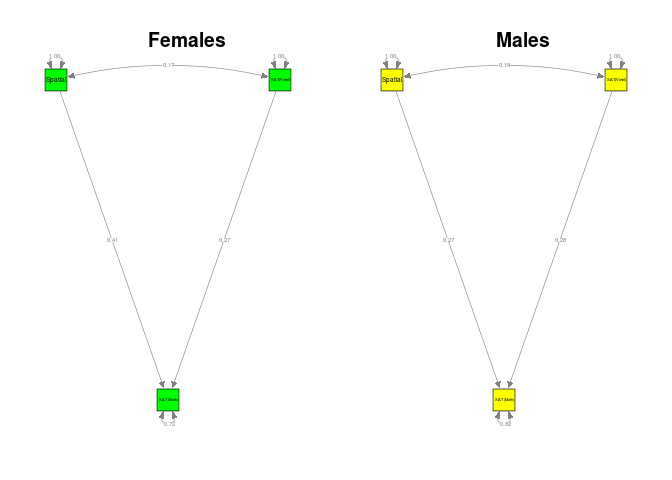## Random-effects TSSEM

### Stage 1 analysis

The $$I^2$$ indices for the correlations between spatial and math, verbal and math, and spatial and verbal are .00, .81 and .23, respectively.

#### Random-effects model
## Stage 1 analysis: A diagonal matrix for random effects
random1 <- tssem1(Becker94$data, Becker94$n, method="REM", RE.type="Diag")
summary(random1)

Call:
meta(y = ES, v = acovR, RE.constraints = Diag(x = paste(RE.startvalues,
"*Tau2_", 1:no.es, "_", 1:no.es, sep = "")), RE.lbound = RE.lbound,
I2 = I2, model.name = model.name, suppressWarnings = TRUE,
silent = silent, run = run)

95% confidence intervals: z statistic approximation
Coefficients:
Estimate   Std.Error      lbound      ubound z value
Intercept1  3.7179e-01  3.6499e-02  3.0025e-01  4.4333e-01 10.1863
Intercept2  4.3173e-01  7.7452e-02  2.7993e-01  5.8354e-01  5.5742
Intercept3  2.0412e-01  4.6874e-02  1.1224e-01  2.9599e-01  4.3545
Tau2_1_1    1.0000e-10  4.7099e-03 -9.2312e-03  9.2312e-03  0.0000
Tau2_2_2    4.8060e-02  2.6340e-02 -3.5652e-03  9.9685e-02  1.8246
Tau2_3_3    5.8428e-03  9.8463e-03 -1.3455e-02  2.5141e-02  0.5934
Pr(>|z|)
Intercept1 < 2.2e-16 ***
Intercept2 2.486e-08 ***
Intercept3 1.333e-05 ***
Tau2_1_1     1.00000
Tau2_2_2     0.06806 .
Tau2_3_3     0.55291
---
Signif. codes:  0 '***' 0.001 '**' 0.01 '*' 0.05 '.' 0.1 ' ' 1

Q statistic on the homogeneity of effect sizes: 83.6582
Degrees of freedom of the Q statistic: 27
P value of the Q statistic: 1.041442e-07

Heterogeneity indices (based on the estimated Tau2):
Estimate
Intercept1: I2 (Q statistic)    0.000
Intercept2: I2 (Q statistic)    0.811
Intercept3: I2 (Q statistic)    0.254

Number of studies (or clusters): 10
Number of observed statistics: 30
Number of estimated parameters: 6
Degrees of freedom: 24
-2 log likelihood: -23.66616
OpenMx status1: 0 ("0" or "1": The optimization is considered fine.
Other values may indicate problems.)
coef(random1, select="fixed")
Intercept1 Intercept2 Intercept3
0.3717902  0.4317340  0.2041150 
coef(random1, select="random")
    Tau2_1_1     Tau2_2_2     Tau2_3_3
0.0000000001 0.0480596685 0.0058428384 

### Stage 2 analysis

## Stage 2 analysis
random2 <- tssem2(random1, Amatrix=A1, Smatrix=S1, diag.constraints=TRUE,
intervals="LB")
summary(random2)

Call:
wls(Cov = pooledS, asyCov = asyCov, n = tssem1.obj\$total.n, Amatrix = Amatrix,
Smatrix = Smatrix, Fmatrix = Fmatrix, diag.constraints = diag.constraints,
cor.analysis = cor.analysis, intervals.type = intervals.type,
mx.algebras = mx.algebras, model.name = model.name, suppressWarnings = suppressWarnings,
silent = silent, run = run)

95% confidence intervals: Likelihood-based statistic
Coefficients:
Estimate Std.Error  lbound  ubound z value
Spatial2Math             0.29600        NA 0.21537 0.37418      NA
Verbal2Math              0.37132        NA 0.21240 0.52949      NA
ErrorVarMath             0.72964        NA 0.58973 0.82994      NA
CorBetweenSpatialVerbal  0.20412        NA 0.11224 0.29599      NA
Pr(>|z|)
Spatial2Math                  NA
Verbal2Math                   NA
ErrorVarMath                  NA
CorBetweenSpatialVerbal       NA

Goodness-of-fit indices:
Value
Sample size                                538.00
Chi-square of target model                   0.00
DF of target model                           0.00
p value of target model                      0.00
Number of constraints imposed on "Smatrix"   1.00
Chi-square of independence model           132.21
DF of independence model                     3.00
RMSEA                                        0.00
RMSEA lower 95% CI                           0.00
RMSEA upper 95% CI                           0.00
SRMR                                         0.00
TLI                                          -Inf
CFI                                          1.00
AIC                                          0.00
BIC                                          0.00
OpenMx status1: 0 ("0" or "1": The optimization is considered fine.
Other values indicate problems.)

We may also plot the models with the labels or the parameter estimates.

## Convert the model to semPlotModel object
my.plot <- meta2semPlot(random2)

## Plot the model with labels
semPaths(my.plot, whatLabels="path", nCharEdges=10, nCharNodes=10, color="red")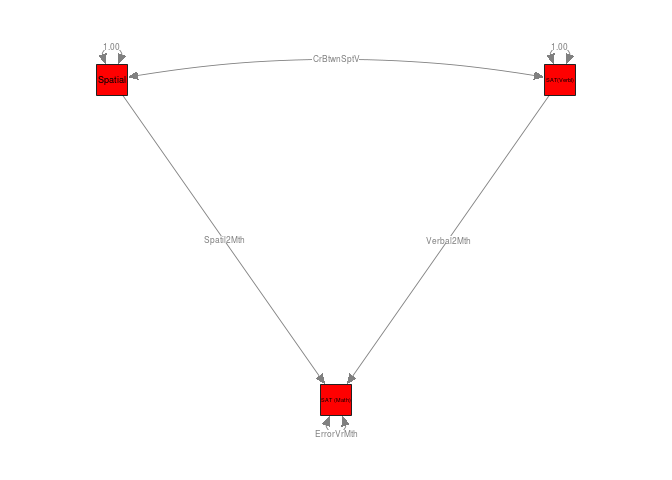## Plot the parameter estimates
semPaths(my.plot, whatLabels="est", nCharNodes=10, color="green")